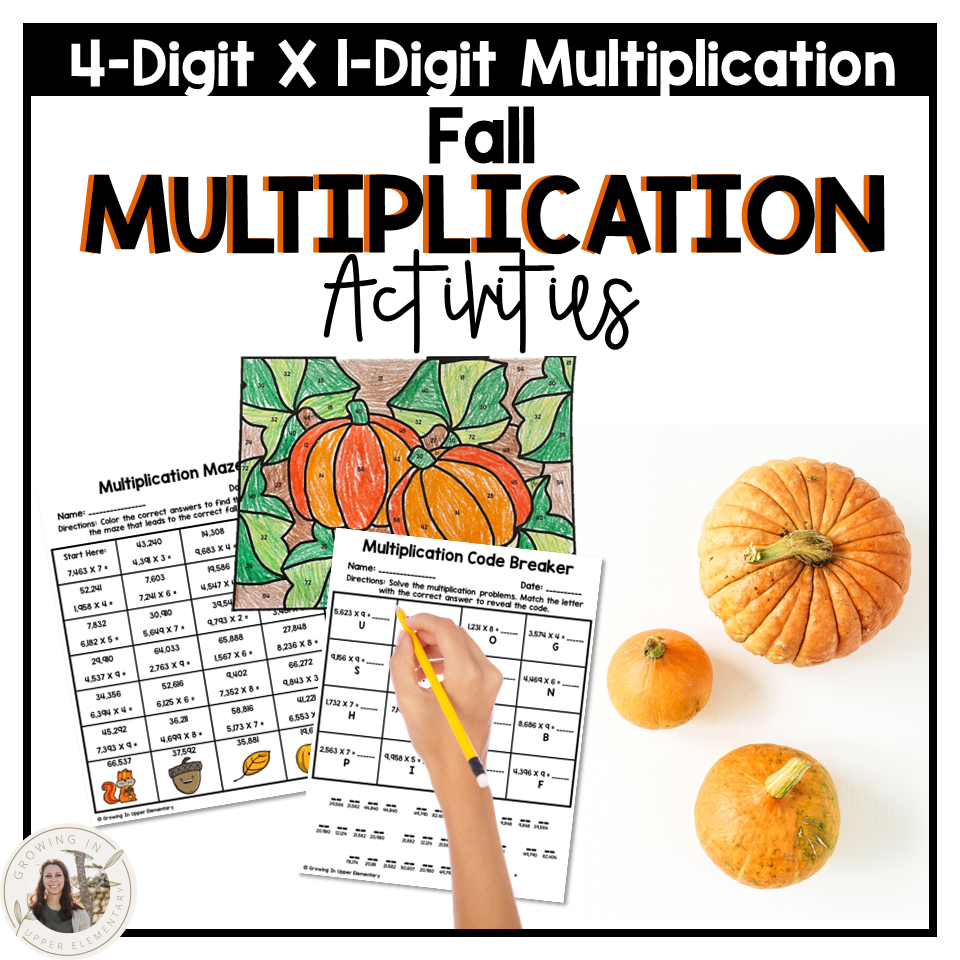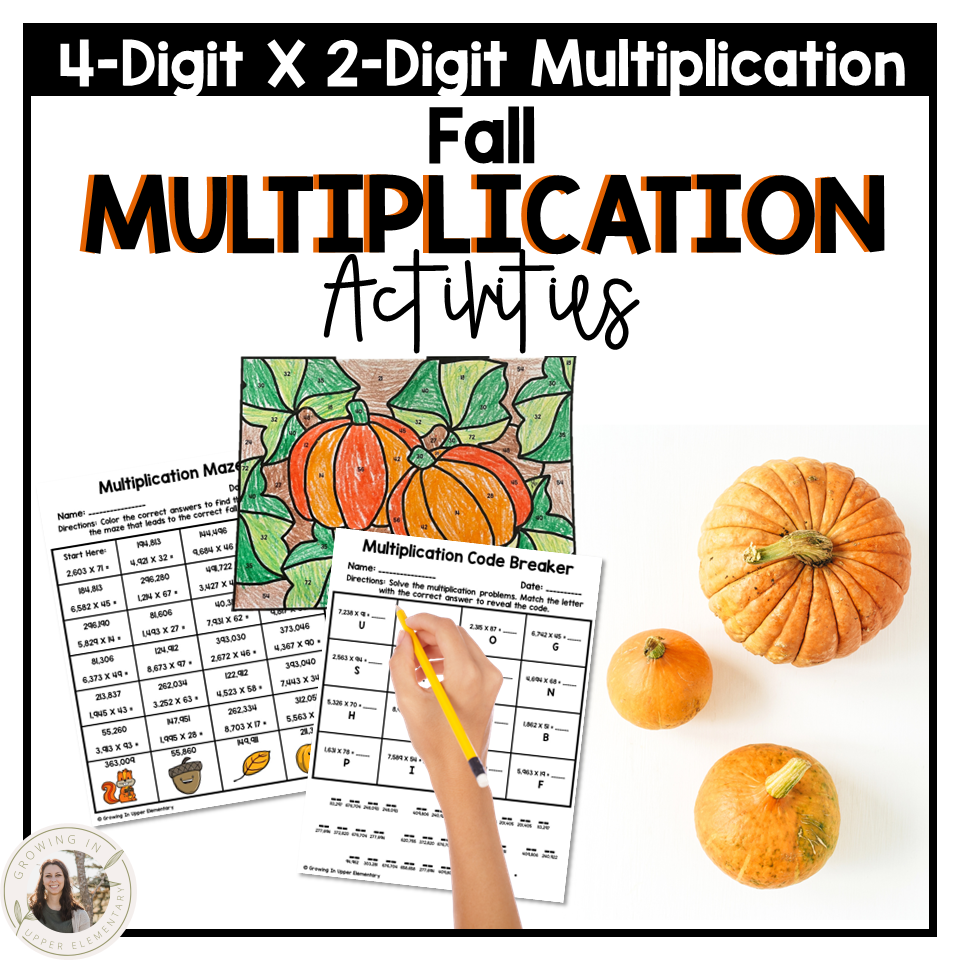top of page
Search
• Saresha Zentner

# Practice Multiplication with these Fun and Engaging Fall Activities

Updated: Oct 24, 2022

Looking for some fun activities to jazz up your math routine while still practicing rigorous multiplication problems? You are in the right place!These engaging activities and unique, easy-to-use, and require no prep from you!

Activities

Each resource has 12 pages of engaging multiplication practice. Here are what activities are included:

Multiplication Color By Code

Students will solve multiplication problems, and then find their answer on the fall coloring page. Then, they will color it accordingly.

Multiplication Code Breaker

Students will solve multiplication problems, and then find which letter corresponds to their answer. The letters form a fall phrase.

Fall Story Problems (2 pages)

Students will estimate an answer to the story problems, show their thinking, and then solve for the answer.

Fall Roll-A-Story Problem (3 pages)

Students will roll a die. Then, they will create a story problem with the given multiplication equation and a fall item that corresponds to the number they rolled.

Multiplication Maze (2 pages)

Students will solve multiplication problems, find the correct answer, and work their way through the multiplication maze.

Multiplication Coloring (2 pages)

Students will solve multiplication problems and then color the fall pictures according to if their answer is even or odd.Sample of Fall Multiplication Activities for 3-Digit X 1-DigitMultiplication Problems

There are three resources to choose from with these fall activities:

Multiplication Facts (2 X 2 through 9 X 9)4-Digit X 2-Digit MultiplicationEasy Differentiation

Use the 4th or 5th grade bundle to make this activity accessible for all students! Simply have students work on the level they are currently at!